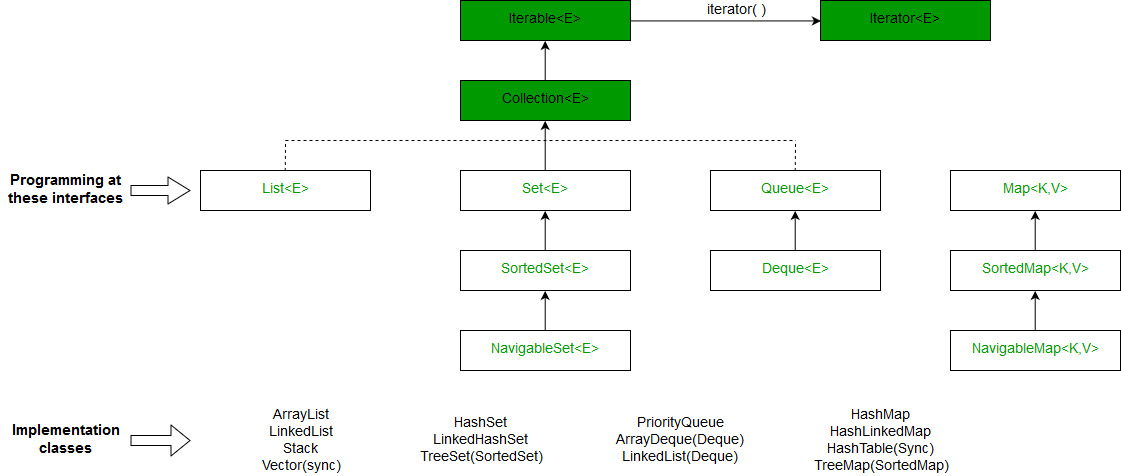Open in App
Not now

# HashMap and TreeMap in Java

• Difficulty Level : Easy
• Last Updated : 15 Dec, 2022

HashMap and TreeMap are part of collection framework.

HashMap

java.util.HashMap class is a Hashing based implementation. In HashMap, we have a key and a value pair<Key, Value>.

` HashMap<K, V> hmap = new HashMap<K, V>();`

Let us consider below example where we have to count occurrences of each integer in given array of integers.

```Input: arr[] = {10, 3, 5, 10, 3, 5, 10};
Output: Frequency of 10 is 3
Frequency of 3 is 2
Frequency of 5 is 2
```

 `/* Java program to print frequencies of all elements using ``   ``HashMap */``import` `java.util.*;`` ` `class` `Main``{``    ``// This function prints frequencies of all elements``    ``static` `void` `printFreq(``int` `arr[])``    ``{``        ``// Creates an empty HashMap``        ``HashMap hmap = ``                     ``new` `HashMap();`` ` `        ``// Traverse through the given array``        ``for` `(``int` `i = ``0``; i < arr.length; i++)``        ``{``            ``Integer c = hmap.get(arr[i]);`` ` `            ``// If this is first occurrence of element ``            ``if` `(hmap.get(arr[i]) == ``null``)``               ``hmap.put(arr[i], ``1``);`` ` `            ``// If elements already exists in hash map``            ``else` `              ``hmap.put(arr[i], ++c);``        ``}`` ` `        ``// Print result``        ``for` `(Map.Entry m:hmap.entrySet())``          ``System.out.println(``"Frequency of "` `+ m.getKey() + ``                             ``" is "` `+ m.getValue());``    ``}`` ` `    ``// Driver method to test above method``    ``public` `static` `void` `main (String[] args)``    ``{``        ``int` `arr[] = {``10``, ``34``, ``5``, ``10``, ``3``, ``5``, ``10``};``        ``printFreq(arr);``    ``}``}`

Output:

```Frequency of 34 is 1
Frequency of 3 is 1
Frequency of 5 is 2
Frequency of 10 is 3```

Key Points

• HashMap does not maintain any order neither based on key nor on basis of value, If we want the keys to be maintained in a sorted order, we need to use TreeMap.
• Complexity: get/put/containsKey() operations are O(1) in average case but we can’t guarantee that since it all depends on how much time does it take to compute the hash.

Application:
HashMap is basically an implementation of hashing. So wherever we need hashing with key value pairs, we can use HashMap. For example, in Web Applications username is stored as a key and user data is stored as a value in the HashMap, for faster retrieval of user data corresponding to a username.

TreeMap

TreeMap can be a bit handy when we only need to store unique elements in a sorted order. Java.util.TreeMap uses a red-black tree in the background which makes sure that there are no duplicates; additionally it also maintains the elements in a sorted order.

` TreeMap<K, V> hmap = new TreeMap<K, V>();`

Below is TreeMap based implementation of same problem. This solution has more time complexity O(nLogn) compared to previous one which has O(n). The advantage of this method is, we get elements in sorted order.

Recommended Practice

 `/* Java program to print frequencies of all elements using ``   ``TreeMap */``import` `java.util.*;`` ` `class` `Main``{``    ``// This function prints frequencies of all elements``    ``static` `void` `printFreq(``int` `arr[])``    ``{``        ``// Creates an empty TreeMap``        ``TreeMap tmap =``                     ``new` `TreeMap();`` ` `        ``// Traverse through the given array``        ``for` `(``int` `i = ``0``; i < arr.length; i++)``        ``{``            ``Integer c = tmap.get(arr[i]);`` ` `            ``// If this is first occurrence of element   ``            ``if` `(tmap.get(arr[i]) == ``null``)``               ``tmap.put(arr[i], ``1``);`` ` `            ``// If elements already exists in hash map``            ``else``              ``tmap.put(arr[i], ++c);``        ``}`` ` `        ``// Print result``        ``for` `(Map.Entry m:tmap.entrySet())``          ``System.out.println(``"Frequency of "` `+ m.getKey() + ``                             ``" is "` `+ m.getValue());``    ``}`` ` `    ``// Driver method to test above method``    ``public` `static` `void` `main (String[] args)``    ``{``        ``int` `arr[] = {``10``, ``34``, ``5``, ``10``, ``3``, ``5``, ``10``};``        ``printFreq(arr);``    ``}``}`

Output:

```Frequency of 3 is 1
Frequency of 5 is 2
Frequency of 10 is 3
Frequency of 34 is 1```

Key Points

• For operations like add, remove, containsKey, time complexity is O(log n where n is number of elements present in TreeMap.
• TreeMap always keeps the elements in a sorted(increasing) order, while the elements in a HashMap have no order. TreeMap also provides some cool methods for first, last, floor and ceiling of keys.

Overview:

1. HashMap implements Map interface while TreeMap implements SortedMap interface. A Sorted Map interface is a child of Map.
2. HashMap implements Hashing, while TreeMap implements Red-Black Tree(a Self Balancing Binary Search Tree). Therefore all differences between Hashing and Balanced Binary Search Tree apply here.
3. Both HashMap and TreeMap have their counterparts HashSet and TreeSet. HashSet and TreeSet implement Set interface. In HashSet and TreeSet, we have only key, no value, these are mainly used to see presence/absence in a set. For above problem, we can’t use HashSet (or TreeSet) as we can’t store counts. An example problem where we would prefer HashSet (or TreeSet) over HashMap (or TreeMap) is to print all distinct elements in an array.Related Articles

This article is contributed by Chirag Agrawal. If you like GeeksforGeeks and would like to contribute, you can also write an article and mail your article to review-team@geeksforgeeks.org. See your article appearing on the GeeksforGeeks main page and help other Geeks.